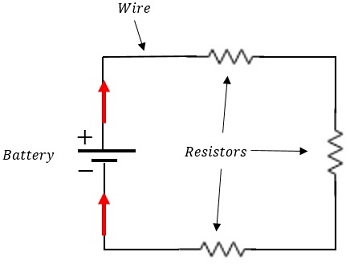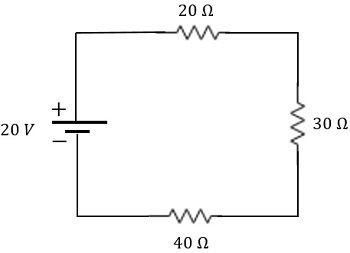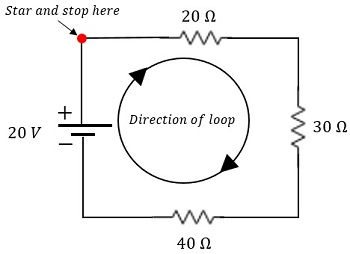Science Courses / Course / Chapter

# Series Circuit: Calculating Voltage Drops with Ohm's Law

Lesson Transcript
Instructor: Matthew Bergstresser

Matthew has a Master of Arts degree in Physics Education. He has taught high school chemistry and physics for 14 years.

In a series circuit, the current flows through each component since there is only one path where the current can flow. Explore the properties of voltages in series circuits and learn how to calculate voltage drops using Ohm's law. Updated: 01/19/2022

## A Roller Coaster Analogy

Imagine that you had to power a cart through a roller coaster by peddling a stationary bicycle. Sounds fun for your friends, but pretty miserable for you! The track starts at a high elevation, goes down hill, and then back up to where it started. Along the way, the track has rough patches resisting the movement of the cart.

This is an analogy for how a series circuit works. The track is the wire, the cart is the current flow, the rough parts of the track are resistors, and you pedaling the bicycle are the battery. Let's flesh out this analogy with an in-depth study of a series circuit.An error occurred trying to load this video.

Try refreshing the page, or contact customer support.

Coming up next: Parallel Circuits: Calculating Currents with Ohm's Law

### You're on a roll. Keep up the good work!

Replay
Your next lesson will play in 10 seconds
• 0:03 A Roller Coaster Analogy
• 0:42 Series Circuits
• 1:56 Ohm's Law &…
• 3:11 Circuit Analysis
• 4:50 Lesson Summary
Save Save

Want to watch this again later?

Timeline
Autoplay
Autoplay
Speed Speed

## Series Circuits

A series circuit is a circuit where electrical current only has one pathway from the positive terminal of a battery, through at least one resistor, and back to the negative terminal of the battery. This diagram shows a basic, series circuit like we just described:The battery pushes the current from the positive terminal through each resistor and back to the negative terminal. In the roller coaster analogy, you wouldn't have to pedal when the cart leaves the top of the roller coaster: gravity does the work for you. Electrical current naturally moves from high potential to low potential too, but it's electrical potential. You and the battery have to do work to make the cart and the current move from low potential back to high potential. This next diagram shows the potential difference of the battery and the resistance of each resistor:When the current flows through a resistor there is a voltage drop. The side of the resistor closest to the high potential side of the battery (the + terminal) has higher potential than the opposite side of the resistor. The voltage drop is caused by the resistor using the electrical energy to do something such as turning a motor. We will use Ohm's law and Kirchhoff's loop rule to determine the voltage drops across the resistors.

## Ohm's Law & Kirchhoff's Loop Rule

Ohm's law is the equation V = IR, where V is for voltage in volts, I is for current in amperes (or amps, or A) and R is for resistance in ohms (or Ω). We can't use this equation alone because we don't know the current through the resistors. This is where Kirchhoff's rule comes in.

Voltage is a change in electric potential. If we start and stop at the same location in a circuit there's no change in voltage. It is like the cart moving through a flat section of the roller coaster track. The change in elevation is zero. In formal physics language, Kirchhoff's loop rule states that in a closed loop, the change in voltage is zero.

We have to trace a path (remember that there's only one) through the circuit and then write the voltage for each resistor and battery along the path. When we move in the direction of current flow, the voltages across the resistors are negative.

Going from the negative terminal of the battery to the positive terminal is an increase in voltage, so it's positive. Adding all of these voltages and setting it equal to zero will allow us to solve for the current in the circuit. Let's analyze our example circuit to determine the change in voltages across each resistor.

## Circuit Analysis

This next diagram shows the loop which we will follow in the circuit analysis:To unlock this lesson you must be a Study.com Member.

### Register to view this lesson

Are you a student or a teacher?

Back

### Resources created by teachers for teachers

Over 30,000 video lessons & teaching resources‐all in one place.Video lessonsQuizzes & WorksheetsClassroom IntegrationLesson Plans

I would definitely recommend Study.com to my colleagues. It’s like a teacher waved a magic wand and did the work for me. I feel like it’s a lifeline.

Jennifer B.
TeacherCreate an account to start this course today
Used by over 30 million students worldwide